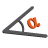Back to Adaptive Vision Library website

You are here: Start » Function Reference » Geometry 2D Angle Metrics

# Geometry 2D Angle Metrics

Select a function from the list below.

Icon Name Description LibraryAngleBetweenDirections Measures the rotation angle from first to second direction. LiteAngleBetweenLines Measures the smaller and the larger angle between two lines. LiteAngleBetweenSegments Measures the angle between two segments with one of four possible metrics. LiteAngleBetweenThreePoints Measures the angle defined by three consecutive points. LiteAngleBetweenVectors Measures the angle between two vectors. LiteNormalizeAngle Normalizes an angle to the range <x, x+180) or <x, x+360). Lite